# [C++]模板初阶与STL简介

1.泛型编程

2.函数模板

3.类模板

4.什么是STL

5.STL的版本

6.STL的六大组件

7.STL的缺陷

## 模板初阶与STL简介：：

### 1.泛型编程

void Swap(int& left, int& right)
{
int temp = left;
left = right;
right = temp;
}
void Swap(double& left, double& right)
{
double temp = left;
left = right;
right = temp;
}
void Swap(char& left, char& right)
{
char temp = left;
left = right;
right = temp;
}


1.重载的函数仅仅是类型不同，代码复用率比较低，只要有新类型出现时，就需要用户自己增加对应的函数

2.代码的可维护性低，一个出错可能所有的重载均出错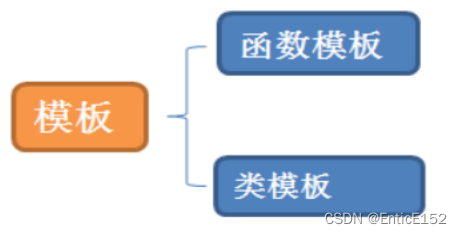### 2.函数模板

template<typename T1, typename T2,......,typename Tn>

template<typename T>
void Swap(T& left, T& right)
{
T temp = left;
left = right;
right = temp;
}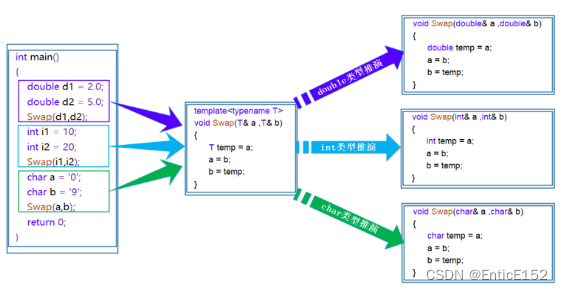1.隐式实例化：让编译器根据实参推演模板参数的实际类型

template<class T>
T Add(const T& left, const T& right)
{
return left + right;
}
int main()
{
int a1 = 10, a2 = 20;
double d1 = 10.0, d2 = 20.0;

/*
该语句不能通过编译，因为在编译期间，当编译器看到该实例化时，需要推演其实参类型
通过实参a1将T推演为int，通过实参d1将T推演为double类型，但模板参数列表中只有一个T，
编译器无法确定此处到底该将T确定为int 或者 double类型而报错
注意：在模板中，编译器一般不会进行类型转换操作，因为一旦转化出问题，编译器就需要背黑锅
*/
// 此时有两种处理方式：1. 用户自己来强制转化 2. 使用显式实例化
return 0;
}

2.显示实例化：在函数名后的<>中指定模板参数的实际类型

int main(void)
{
int a = 10;
double b = 20.0;
// 显式实例化
return 0;
}  

1.一个非模板函数可以和一个同名的模板函数同时存在，而且该函数模板还可以被实例化为这个非模板函数。

// 专门处理int的加法函数
{
return left + right;
}
// 通用加法函数
template<class T>
{
return left + right;
}
void Test()
{
}

2.对于非模板函数和同名函数模板，如果其他条件都相同，在调用时会优先调用非模板函数而不会从该模板产生出一个实例。如果模板可以产生一个具有更好匹配的函数，那么将选择模板。

// 专门处理int的加法函数
{
return left + right;
}
// 通用加法函数
template<class T1, class T2>
{
return left + right;
}
void Test()
{
}

3.模板函数不允许自动类型转换，但普通函数可以进行自动类型转换。

### 3.类模板

typedef int STDataType;
class StackInt
{
public:
Stack(int capacity = 4)
{
cout << "Stack(int capacity = )" << capacity << endl;
_a = (STDataType*)malloc(sizeof(STDataType) * capacity);
if (_a == nullptr)
{
perror("malloc fail");
exit(-1);
}
}
~Stack()
{
cout << "~Stack()" << endl;
free(_a);
_a = nullptr;
_top = _capacity = 0;
}
void Push(STDataType x)
{
//...
//扩容
_a[_top++] = x;
}
private:
STDataType* _a;
int _top;
int _capacity;
};

typedef double STDataType;
class StackDouble
{
public:
Stack(int capacity = 4)
{
cout << "Stack(int capacity = )" << capacity << endl;
_a = (STDataType*)malloc(sizeof(STDataType) * capacity);
if (_a == nullptr)
{
perror("malloc fail");
exit(-1);
}
}
~Stack()
{
cout << "~Stack()" << endl;
free(_a);
_a = nullptr;
_top = _capacity = 0;
}
void Push(STDataType x)
{
//...
//扩容
_a[_top++] = x;
}
private:
STDataType* _a;
int _top;
int _capacity;
};

当我们需要两个栈，一个在栈中插入int类型的数据，一个在栈中插入double类型的数据，那么typedef将不再适用，代码可重复性过高。

template<typename T>
class Stack
{
public:
Stack(int capacity = 4)
{
cout << "Stack(int capacity = )" << capacity << endl;
_a = (T*)malloc(sizeof(T) * capacity);
if (_a == nullptr)
{
perror("malloc fail");
exit(-1);
}
}
~Stack()
{
cout << "~Stack()" << endl;
free(_a);
_a = nullptr;
_top = _capacity = 0;
}
void Push(const T& x)
{
//...
//扩容
_a[_top++] = x;
}
private:
T* _a;
int _top;
int _capacity;
};
int main()
{
//类模板没有推演的时机，函数模板通过实参传递形参，推演模板参数
//类模板统一显示实例化
//他们是同一个类模板实例化出来的 但是模板参数不同 他们就是不同的类
Stack<int> st1;
st1.Push(1);
Stack<double> st2;
st2.Push(1.1);
return 0;
}

template<class T1, class T2, ..., class Tn>
class 类模板名
{
// 类内成员定义
}；
// 动态顺序表
// 注意：Vector不是具体的类，是编译器根据被实例化的类型生成具体类的模具
template<class T>
class Vector
{
public:
Vector(size_t capacity = 10)
: _pData(new T[capacity])
, _size(0)
, _capacity(capacity)
{}
// 使用析构函数演示：在类中声明，在类外定义。
~Vector();
void PushBack(const T& data)；
void PopBack()；
// ...
size_t Size()
{
return _size;
}
T& operator[](size_t pos)
{
assert(pos < _size);
return _pData[pos];
}
private:
T* _pData;
size_t _size;
size_t _capacity;
};
//注意：类模板中函数放在类外进行定义时，需要加模板参数列表template<class T>
template <class T>
Vector<T>::~Vector()
{
if (_pData)
{
delete[] _pData;
}
_size = _capacity = 0;
}

// Vector类名，Vector<int>才是类型
Vector<int> s1;
Vector<double> s2;

相比静态数组的优势 越界的检查能检查出来(C语言的静态数组对于越界的检查是抽查 越界写可能检查到 越界读检查不到)
#define N 10
template<class T>
class array
{
public:
inline T& operator[](size_t i)
{
assert(i < N);
return _a[i];
}
private:
T _a[N];
};
int main()
{
array<int> a1;
for (size_t i = 0; i < N; ++i)
{
//等价于a1.operator[](i) = i
a1[i] = i;
}
for (size_t i = 0; i < N; ++i)
{
a1[i]++;
}
for (size_t i = 0; i < N; ++i)
{
cout << a1[i] << endl;
}
return 0;
}

1.在定义的地方显示实例化
2.模板函数不声明和定义到两个文件.h和.cpp中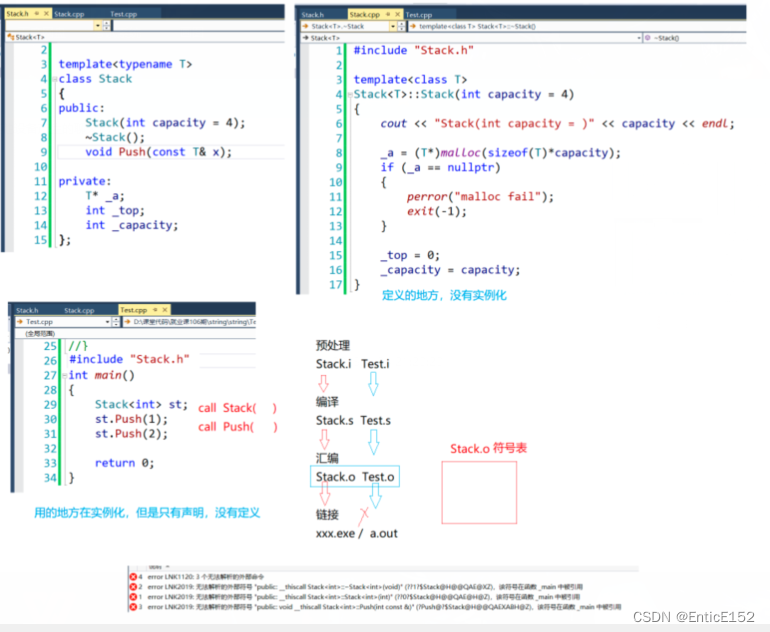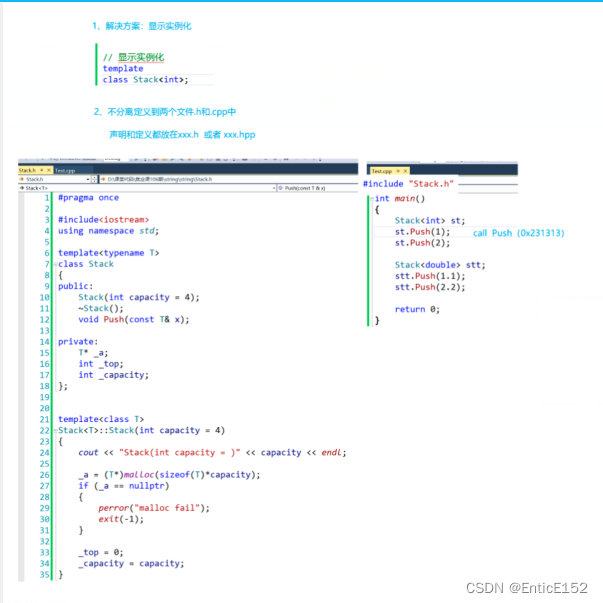### 4.什么是STL

STL(standard template libaray—标准模板库)：是C++标准库的重要组成部分，不仅是一个可复用的组件库，而且是一个包含数据结构与算法的软件框架。

### 5.STL的版本

Alexander Stepanov、Meng Lee在惠普实验室完成的原始版本，本着开源的精神，他们声明允许任何人任意运用、拷贝、修改、传播、商用这些代码，无需付费。唯一的条件就是需要向原始版本一样做开源使用。HP版本—所有STL实现版本的始祖。

P.J.版本：

RW版本：

SGI版本：

### 6.STL的六大组件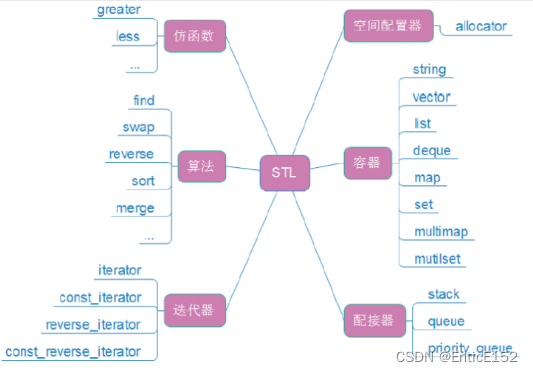### 7.STL的缺陷

1.STL库的更新太慢了，上一版是C++98，中间的C++03做了一些修订，C++11出来已经相隔13年了，STL才进一步更新。

2.STL现在都没有支持线程安全，并发环境下需要我们自己加锁，并且锁的粒度是比较大的。

3.STL极度的追求效率，导致内部比较复杂。比如类型萃取，迭代器萃取。

4.STL的使用会有代码膨胀的问题，比如使用vector/vector/vector这样会生成多份代码，当然这是模板语法本身导致的。

08-2343
02-2893
09-15748
11-08214
03-1688
12-17278
02-111332
07-24556
03-051290
09-0682
12-30556
11-0886
10-31204
02-24685
10-17124
05-16438
08-0824
03-30106

### “相关推荐”对你有帮助么？

•非常没帮助
•没帮助
•一般
•有帮助
•非常有帮助被折叠的  条评论 为什么被折叠?到【灌水乐园】发言EnticE152

¥1 ¥2 ¥4 ¥6 ¥10 ¥20获取中扫码支付点击重新获取扫码支付1.余额是钱包充值的虚拟货币，按照1:1的比例进行支付金额的抵扣。
2.余额无法直接购买下载，可以购买VIP、付费专栏及课程。余额充值